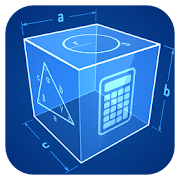# Geometry - Lumos Educational App Store4.51
Price -fREE
\$0

#### DESCRIPTION:

Geometric Calculator Calculates plane and solid figures: Triangle, square, rectangle, parallelogram, rhombus, trapezoid, rectangle, polygon, circle, circle, ellipse. Sphere, cube, box, cylinder, cone, truncated cone, prism, pyramid, truncated pyramid, the octahedron. For each shape type there are solution formulas and solutions itself based on an input data! Planimetrics ~~~~~~~~~ Triangle - Parties - Angles - Area - Perimeter Square - Parties - Diagonal - Area - Perimeter Rectangle - Diagonal - Area - Perimeter Parallelogram - Diagonals - Area - Perimeter

#### OVERVIEW:

Geometry is a free educational mobile app By .It helps students in grades HS practice the following standards HSG.CO.A.3.

This page not only allows students and teachers download Geometry but also find engaging Sample Questions, Videos, Pins, Worksheets, Books related to the following topics.

1. HSG.CO.A.3 : Given a rectangle, parallelogram, trapezoid, or regular polygon, describe the rotations and reflections that carry it onto itself..

HS

HSG.CO.A.3

#### ADDITIONAL INFORMATION:

Developer:

Software Version: 2.6

Category:

### RELATED APPSEdSearch WebSearch